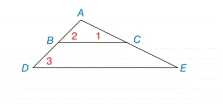Chapter 2.3, Problem 17E### Elementary Geometry for College St...

6th Edition
Daniel C. Alexander + 1 other
ISBN: 9781285195698

#### Solutions

Chapter
Section### Elementary Geometry for College St...

6th Edition
Daniel C. Alexander + 1 other
ISBN: 9781285195698
Textbook Problem
1 views

# In Exercise 17 and 18 , complete each proof by filling in the missing statements and reasons. Given: ∠ 1 and ∠ 2 are complementary ∠ 3 and ∠ 1 are complementary Prove: B C ¯ ∥ D E ¯To determine

To check:

The given statement.

Explanation

Given:

The given statement is;

3 and 1 are complementary.

1 and 2 are complementary.

Figure (1)

Approach:

(1) If two lines are cut by a transversal so that two corresponding angles are congruent, then these lines are parallel.

(2) Complementary angles add up to 90°

Approach:

The given statement is;

3 and 1 are complementary.

m3+m1=90°m3=90°m1

1 and 2 are complementary.

### Still sussing out bartleby?

Check out a sample textbook solution.

See a sample solution

#### The Solution to Your Study Problems

Bartleby provides explanations to thousands of textbook problems written by our experts, many with advanced degrees!

Get Started

#### If f(x)=0sinx1+t2dt and g(y)=3yf(x)dx, find g(/6).

Single Variable Calculus: Early Transcendentals, Volume I

#### Let f(x) = 3x2 + 5x 2. Find: a. f(2) b. f(a + 2) c. f(2a) d. f(a + h)

Applied Calculus for the Managerial, Life, and Social Sciences: A Brief Approach

#### True or False: f(x) = 4x2 8x + 1 is increasing on (0, 1).

Study Guide for Stewart's Single Variable Calculus: Early Transcendentals, 8th